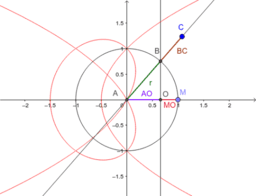# How to use the LocusEquation command?

This book is an introduction to the LocusEquation command in GeoGebra, available since version 4.2. Its target audience is teachers who work in secondary schools. To compute locus equations, efficient algorithms may be required. Since version 4.4 such equations can be quickly displayed not only in desktop mode in GeoGebra, but also in a web browser (even on tablets). There are, however, some tricks which are important to know. This step-by-step howto helps in technical questions primarily, but the demo applets can be further developed to be full featured student applets as well.
•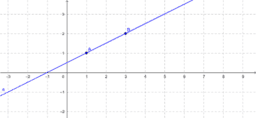### Linear locus equation

•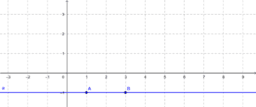### Quadratic locus equation

•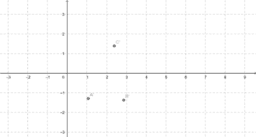### Quadratic locus equation with dynamic coordinates

•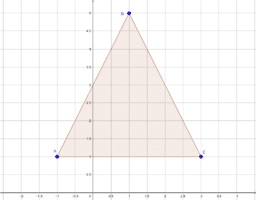### Discovering the Simson-Wallace theorem

•